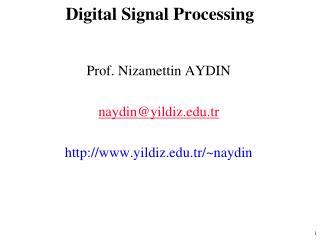DownloadDownload PresentationDigital Signal Processing

# Digital Signal Processing

Download Presentation## Digital Signal Processing

- - - - - - - - - - - - - - - - - - - - - - - - - - - E N D - - - - - - - - - - - - - - - - - - - - - - - - - - -
##### Presentation Transcript

1. Digital Signal Processing Prof. Nizamettin AYDIN naydin@yildiz.edu.tr http://www.yildiz.edu.tr/~naydin

2. Digital Signal Processing Lecture 20 Fourier Transform Properties

3. READING ASSIGNMENTS • This Lecture: • Chapter 11, Sects. 11-5 to 11-9 • Tables in Section 11-9 • Other Reading: • Recitation: Chapter 11, Sects. 11-1 to 11-9 • Next Lectures: Chapter 12 (Applications)

4. LECTURE OBJECTIVES • The Fourier transform • More examples of Fourier transform pairs • Basic properties of Fourier transforms • Convolution property • Multiplication property

5. Fourier Transform Fourier Synthesis (Inverse Transform) Fourier Analysis (Forward Transform)

6. WHY use the Fourier transform? • Manipulate the “Frequency Spectrum” • Analog Communication Systems • AM: Amplitude Modulation; FM • What are the “Building Blocks” ? • Abstract Layer, not implementation • Ideal Filters: mostly BPFs • Frequency Shifters • aka Modulators, Mixers or Multipliers: x(t)p(t)

7. Frequency Response • Fourier Transform of h(t) is the Frequency Response

8. Table of Fourier Transforms

9. Fourier Transform of a General Periodic Signal • If x(t) is periodic with period T0 ,

10. Square Wave Signal

11. Square Wave Fourier Transform

12. Table of Easy FT Properties Linearity Property Delay Property Frequency Shifting Scaling

13. Scaling Property

14. Scaling Property

15. Uncertainty Principle • Try to make x(t) shorter • Then X(jw) will get wider • Narrow pulses have wide bandwidth • Try to make X(jw) narrower • Then x(t) will have longer duration • Cannot simultaneously reduce time duration and bandwidth

16. Significant FT Properties Differentiation Property

17. Convolution Property • Convolution in the time-domain corresponds to MULTIPLICATION in the frequency-domain

18. Convolution Example • Bandlimited Input Signal • “sinc” function • Ideal LPF (Lowpass Filter) • h(t) is a “sinc” • Output is Bandlimited • Convolve “sincs”

19. Ideally Bandlimited Signal

20. Convolution Example

21. Cosine Input to LTI System

22. Ideal Lowpass Filter

23. Ideal Lowpass Filter

24. Signal Multiplier (Modulator) • Multiplication in the time-domain corresponds to convolution in the frequency-domain.

25. Frequency Shifting Property

26. Differentiation Property Multiply by jw